## Tuesday, 12 October 2021

### The Parallel Propagator and using it on the surface of a sphere

The three page Appendix I is on the parallel propagator which gives a general solution to the parallel transport equation of a vector. The appendix shows how to get this from the parallel transport equation which is, for a vector $V^\nu$ transported along a curve $x^\mu\left(\lambda\right)$ $$\frac{dx^\mu}{d\lambda}\partial_\mu V^\nu+\frac{dx^\mu}{d\lambda}\Gamma_{\mu\sigma}^\nu V^\sigma=0$$First we note that the transported vector can be calculated by$$V^\mu\left(\lambda\right)=P_{\ \ \rho}^\mu\left(\lambda,\lambda_0\right)V^\rho\left(\lambda_0\right)$$where $V^\rho\left(\lambda_0\right)$ is the vector at the start point $x^\mu\left(\lambda_0\right)$ and $P_{\ \ \rho}^\mu\left(\lambda,\lambda_0\right)$ is some matrix which is called the parallel propagator. Next we define another matrix $$A_{\ \ \rho}^\mu\left(\lambda\right)=-\Gamma_{\sigma\rho}^\mu\frac{dx^\sigma}{d\lambda}$$and then show that (dropping indices on the matrices)$$P\left(\lambda,\lambda_0\right)=I+\sum_{n=1}^{n=\infty}T_n$$where $I$ is the identity matrix and $$T_n=\int_{\lambda_0}^{\lambda}{\int_{\lambda_0}^{\eta_n}\int_{\lambda_0}^{\eta_{n-1}}{\ldots\int_{\lambda_0}^{\eta_2}A\left(\eta_n\right)A\left(\eta_{n-1}\right)\ldots A\left(\eta_1\right)}d^n\eta}$$$$=\frac{1}{n!}\int_{\lambda_0}^{\lambda}{\int_{\lambda_0}^{\lambda}\int_{\lambda_0}^{\lambda}{\ldots\int_{\lambda_0}^{\lambda}\mathcal{P}\left[A\left(\eta_n\right)A\left(\eta_{n-1}\right)\ldots A\left(\eta_1\right)\right]}d^n\eta}$$where $\mathcal{P}$ orders the matrices $A\left(\eta_i\right)$ so that $\eta_n\geq\eta_{n-1}\geq\ldots\geq\eta_1$.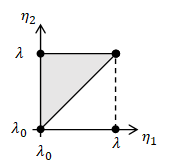2-simplex

The first integral is over $n$-dimensional equilateral right triangles, or $n$-simplices and is quite hard to calculate but $n!$ $n$-simplices make an $n$-cube which makes the second integral which is much easier to calculate. I had a bit of trouble getting my head round all that and I tested it on a few examples including vectors transported along lines of constant latitude.  It all works!

### Vectors transported round latitudes calculated by parallel propagator

Note vector length never changes. $T_n$ was evaluated to 5 decimal places.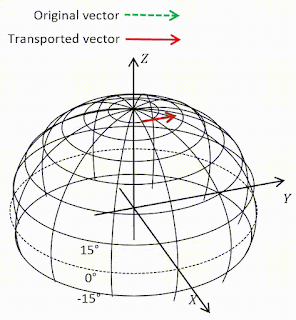Transport at 80°N. Vector barely changes because it's nearly flat up there.$T_n=0$ at $n=25$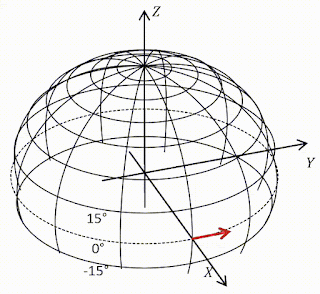Transport round equator. Vector remains parallel because equator is geodesic.$T_n=0$ at $n=1$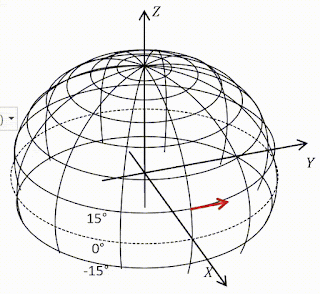Transport at 15°N. Vector rotates down by 87°.$T_n=0$ at $n=11$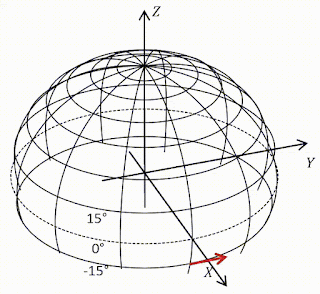Transport at 15°S.Vector rotates up by 87°.$T_n=0$ at $n=11$